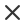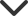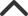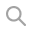Hello, Guest
Languages
EnglishFILTER# belchonoksun

## 1,582 Royalty free images, vectors, footage and audio filesConnect with belchonoksunMath vector technical seamless pattern with handwritten formulas, calculations, plots, signs, equations, shuffled together on a green blackboard backgroundPREMIUMMathematical vector endless seamless pattern with formulas, figures and calculations handwritten on the background of stars. Scientific space endless texturePREMIUMMath vector seamless texture with various mathematical signs, calculations, formulas, equations, figures. Algebraic endless pattern, handwritten on a grid copybook paperPREMIUMChemical seamless pattern with formulas, schemes and equations. Scientific endless texture. You can use any color of backgroundPREMIUMMulticolored mathematical vector seamless pattern with geometrical plots, formulas and calculations. Math colorful endless texture. You can use any color of backgroundPREMIUMAlgebraic vector seamless texture with many various mathematical signs, calculations, formulas. Math endless pattern, handwritten on a grid copybook paperPREMIUMMulticolored mathematical vector seamless pattern with geometrical plots, formulas and calculations. Math colorful endless texture. You can use any color of backgroundPREMIUMScientific vector endless texture with chemical and physical figures, plots, formulas, equations and question marks. Physics seamless pattern. You can use any color of backgroundPREMIUMEndless mathematical texture with geometrical figures, plots and equations, handwritten with chalk on a grey blackboard. Mathematical vector seamless patternPREMIUMEndless food vector texture with coffee cup, curls and words "Coffee" handwritten by chalk on grey board. Food and drink vector seamless patternPREMIUMFood and drink vector seamless pattern with various coffee cups and words "Coffee" handwritten by chalk on grey board. Endless food vector texturePREMIUMEndless food and drink texture with tea cups, teapots, tea leaves and handwritten words "tea", handwritten by chalk on grey board, vectorPREMIUMScientific vector seamless texture with equations and formulas of chemical combinations, "handwritten on a grid copybook paper"PREMIUMMath vector seamless texture with formulas, plots, functions, equations and algebraic and geometric figures, "handwritten on a grid copybook paper"PREMIUMMath education vector seamless pattern with handwritten geometrical figures, computing, formulas, plots and tasks. Endless texture. Mathematical background handwritten on a copybook grid paperPREMIUMScientific vector seamless pattern with math and physical formulas, chemistry plots and graphic schemes, shuffled together. Endless math texture. You can use any color of backgroundPREMIUMMath vector seamless texture with formulas, plots, algebraic and geometric figures and functions, "handwritten on a grid copybook paper"PREMIUMVector seamless endless mathematical texture with math and physical figures, plots and formulas, "handwritten on the old copybook paper"PREMIUMMath education vector seamless pattern with figures, handwritten formulas, tasks, plots and calculations. Mathematical background handwritten on a copybook grid paper, endless texturePREMIUMPhysical vector seamless pattern with the equations, figures, schemes, plots and other calculations, handwritten texture on a copybook paper sheetPREMIUMMathematical vector seamless texture with figures, equations and calculations. Endless math texture, "handwritten on copybook paper sheet"PREMIUMPhysical vector seamless pattern with the equations, figures, schemes, plots and other calculations. You can use any color of backgroundPREMIUMMathematical vector seamless pattern with geometrical figures, trigonometry plots and equations, "handwritten on the grid copybook paper". Endless math texturePREMIUMMathematical vector seamless pattern with figures, formulas and other calculations, "handwritten on the copybook paper", different colors. Endless math texture. You can use any color of backgroundPREMIUMMathematical vector seamless pattern with geometrical figures, plots and equations, "handwritten on the grid copybook paper". Endless math texturePREMIUMMathematical vector seamless pattern with geometrical figures, plots and equations, "handwritten on the grid copybook paper". Endless math texturePREMIUMMathematical vector seamless pattern with geometrical plots, figures, equations, formulas and calculations. Endless math texture. You can use any color of backgroundPREMIUMProgramming technical seamless pattern with programming code program flow diagrams formulas technical devices and schemes. Endless computer texture. You can use any color of backgroundPREMIUMSpace vector seamless pattern with planets and satellites, stars and constellations. Endless texture. You can use any color of backgroundPREMIUMMathematical educational vector seamless pattern with geometrical figures, plots and equations, \"handwritten on the grid copybook paper\". Endless math texturePREMIUMMathematical vector seamless pattern with geometrical figures, plots and equations, \"handwritten on the grid copybook paper\". Endless math texturePREMIUMMath education vector seamless pattern with handwritten formulas, tasks, plots, calculations and geometrical figures. Endless texture. Mathematical background handwritten on a copybook grid paperPREMIUMBeautiful educational chemistry vector seamless pattern with plots, formulas and laboratory equipment. Scientific background. Endless texturePREMIUMMathematical vector seamless pattern with geometrical figures and formulas, \\\"handwritten on a grid copybook paper\\\". Endless texture.PREMIUMMathematical vector seamless pattern with geometrical figures, plots and formulas, \\\"handwritten on a grid copybook paper\\\"PREMIUMBeautiful vector seamless pattern with mathematical figures, formulas and symbols, \\\\\\\\\\\\\\\"handwritten on the copybook paper\\\\\\\\\\\\\\\". You can use any color of backgroundPREMIUMBeautiful vector seamless pattern with mathematical figures, calculations and plots, \\\\\\\\\\\\\\\\\\\\\\\\\\\\\\\"handwritten on the copybook paper\\\\\\\\\\\\\\\\\\\\\\\\\\\\\\\"PREMIUMVector seamless math pattern with mathematical figures, calculations and plots, \\\\\\\\\\\\\\\\\\\\\\\\\\\\\\\"handwritten on the white paper\\\\\\\\\\\\\\\\\\\\\\\\\\\\\\\"PREMIUMChemistry vector seamless pattern with formulas, calculations and laboratory equipment, \"I love chemistry\", \"handwritten on a grid copybook paper\"PREMIUMMathematical vector seamless pattern with many question marks and other mathematical calculations, \"handwritten on the copybook paper\"PREMIUMMathematical vector seamless pattern with figures, formulas and equations, \\\"handwritten on the copybook paper\\\". You can use any color of backgroundPREMIUMBeautiful vector seamless pattern with mathematical figures and formulas, \\\\\\\"handwritten on the old copybook paper\\\\\\\"PREMIUMBeautiful vector seamless pattern with mathematical figures and plots, \\\\\\\"handwritten on the old copybook paper\\\\\\\"PREMIUM